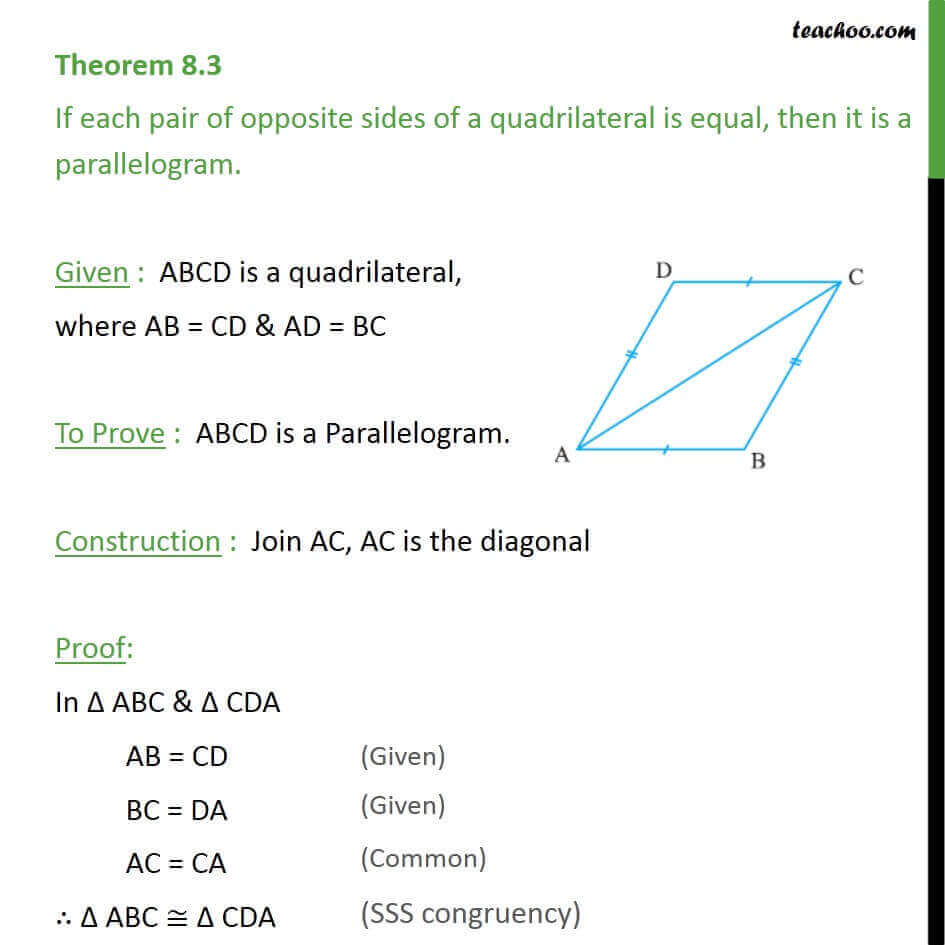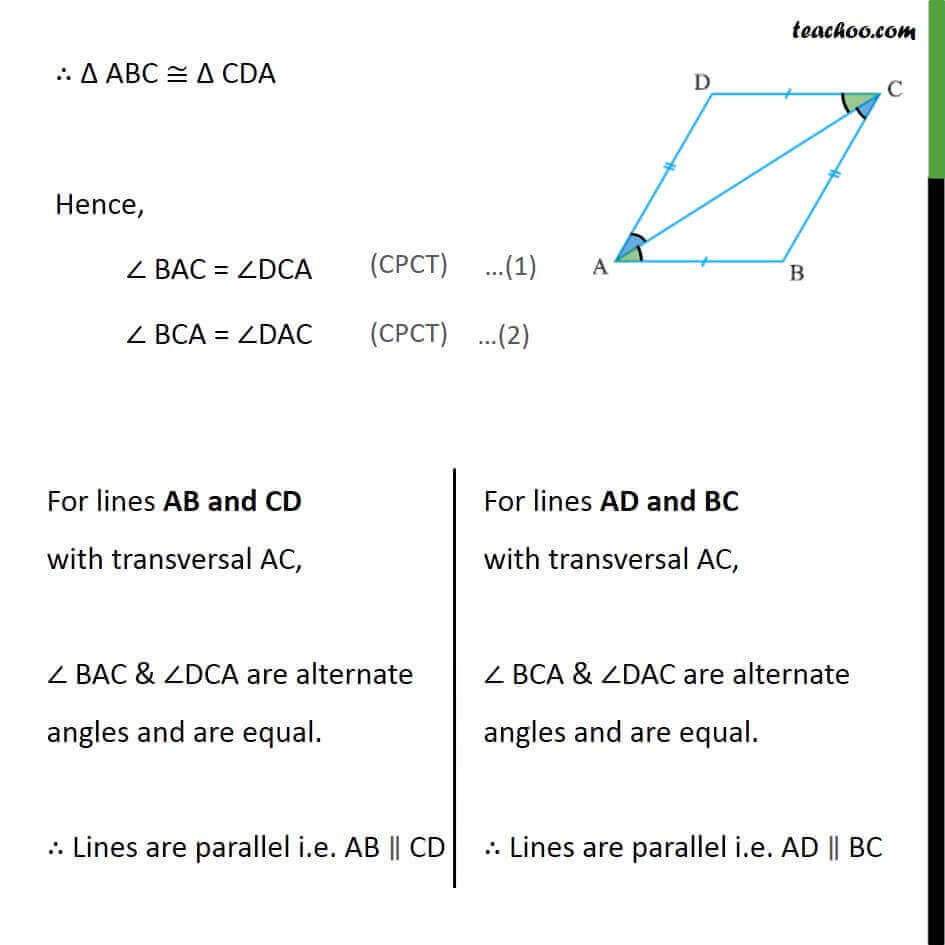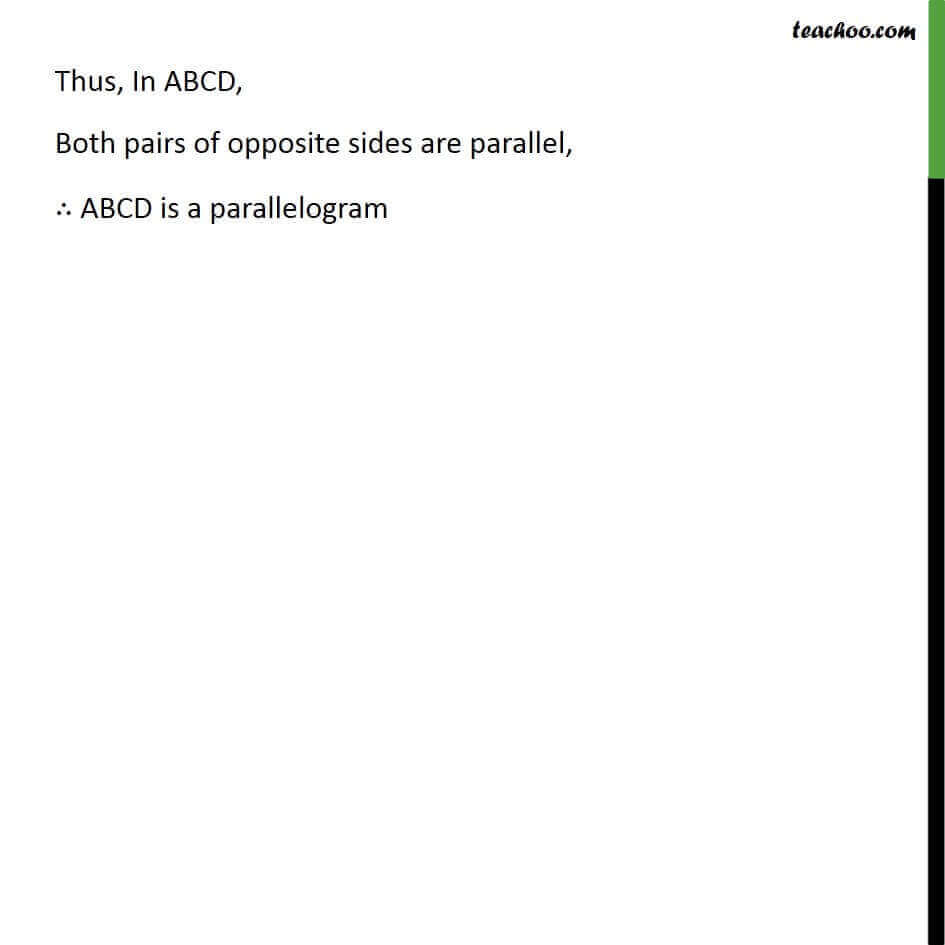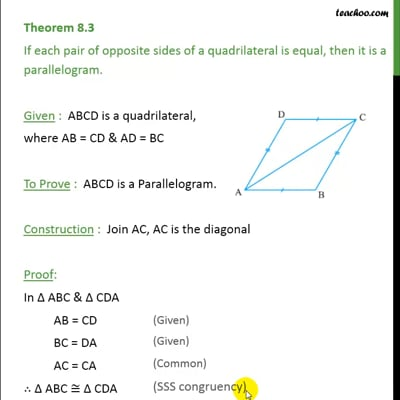Theorems

Chapter 8 Class 9 Quadrilaterals
Serial order wiseThis video is only available for Teachoo black users

Solve all your doubts with Teachoo Black (new monthly pack available now!)

### Transcript

Theorem 8.3 If each pair of opposite sides of a quadrilateral is equal, then it is a parallelogram. Given : ABCD is a quadrilateral, where AB = CD & AD = BC To Prove : ABCD is a Parallelogram. Construction : Join AC, AC is the diagonal Proof: In Δ ABC & Δ CDA AB = CD BC = DA AC = CA ∴ Δ ABC ≅ Δ CDA ∴ Δ ABC ≅ Δ CDA Hence, ∠ BAC = ∠DCA ∠ BCA = ∠DAC Thus, In ABCD, Both pairs of opposite sides are parallel, ∴ ABCD is a parallelogram